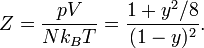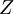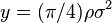# Henderson hard disk equation of state

Henderson hard disk equation of state (Eq. 5 ):$Z = \frac{ p V}{N k_B T} = \frac{ 1 + y^2/8 }{(1-y)^2 }.$

where:

•$Z$ is the compressibility factor
•$p$ is the pressure
•$V$ is the volume
•$N$ is the number of particles
•$k_B$ is the Boltzmann constant
•$T$ is the absolute temperature
•$y= (\pi/4)\rho \sigma^2$